## Packet Power Blog

Few things seem to cause as much confusion as three-phase power, especially in a Delta configuration.  Plumbing and car enthusiasts: rejoice! In this post we'll present a plumber's (and car mechanic's) version of a three phase power system.

Imagine an alternating current water system that delivers alternating pulses of water pressure and vacuum in a closed loop system, using two pipes. Water flows to the receiver (some sort of a hydraulic engine) through one pipe (lets call it A), then back to the source through another pipe (lets call it N). Every few seconds the direction of water flow reverses.  You can imagine the two pipes going to two ends of a cylinder, pushing and pulling a piston in a single-cylinder engine, translating the pulses of water into useful work.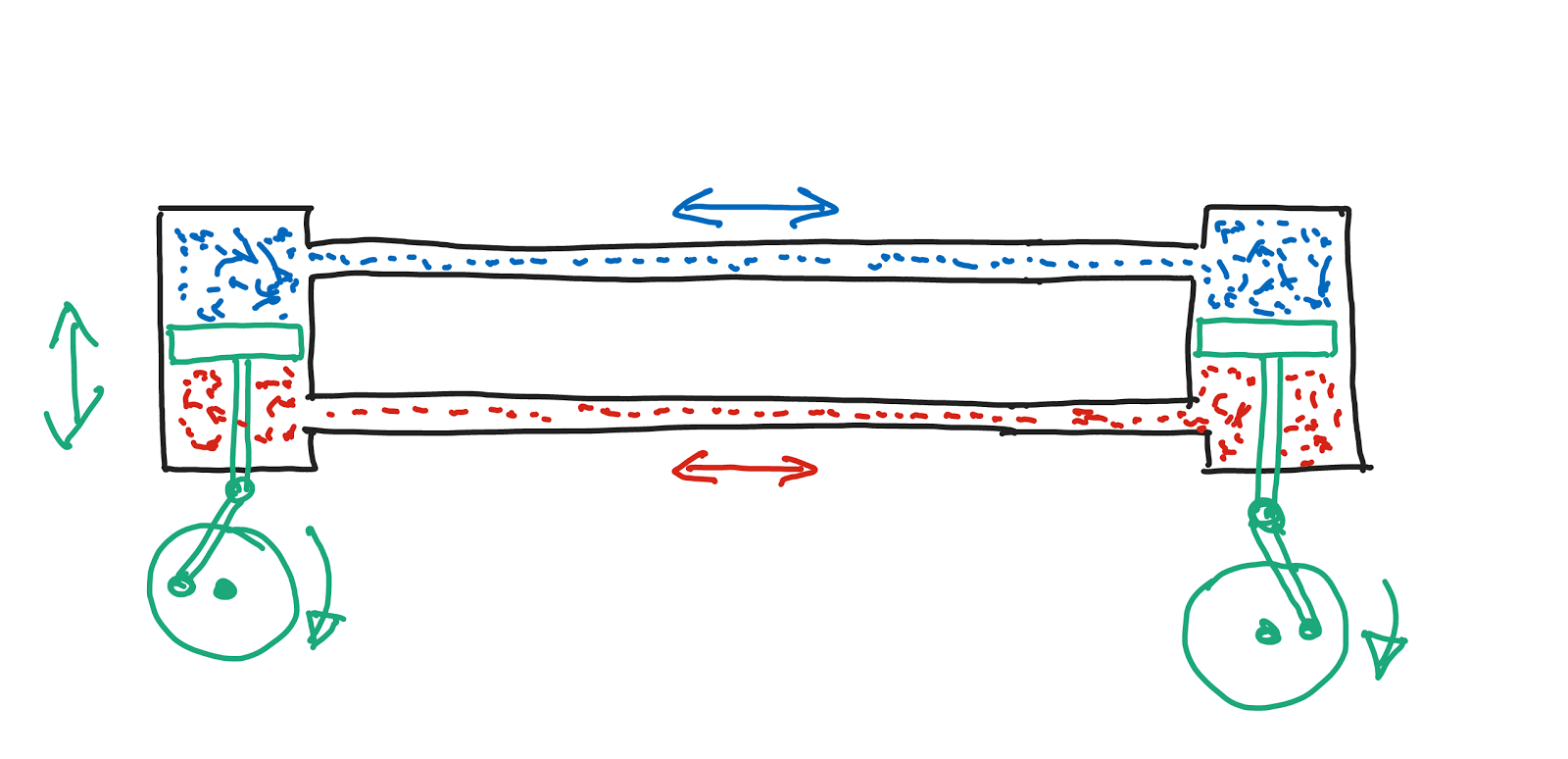Alternating current water system

Now imagine that you wanted to deliver three times as much power. You would need three such systems (A, B and C, with six pipes total, A-N1, B-N2 and C-N3).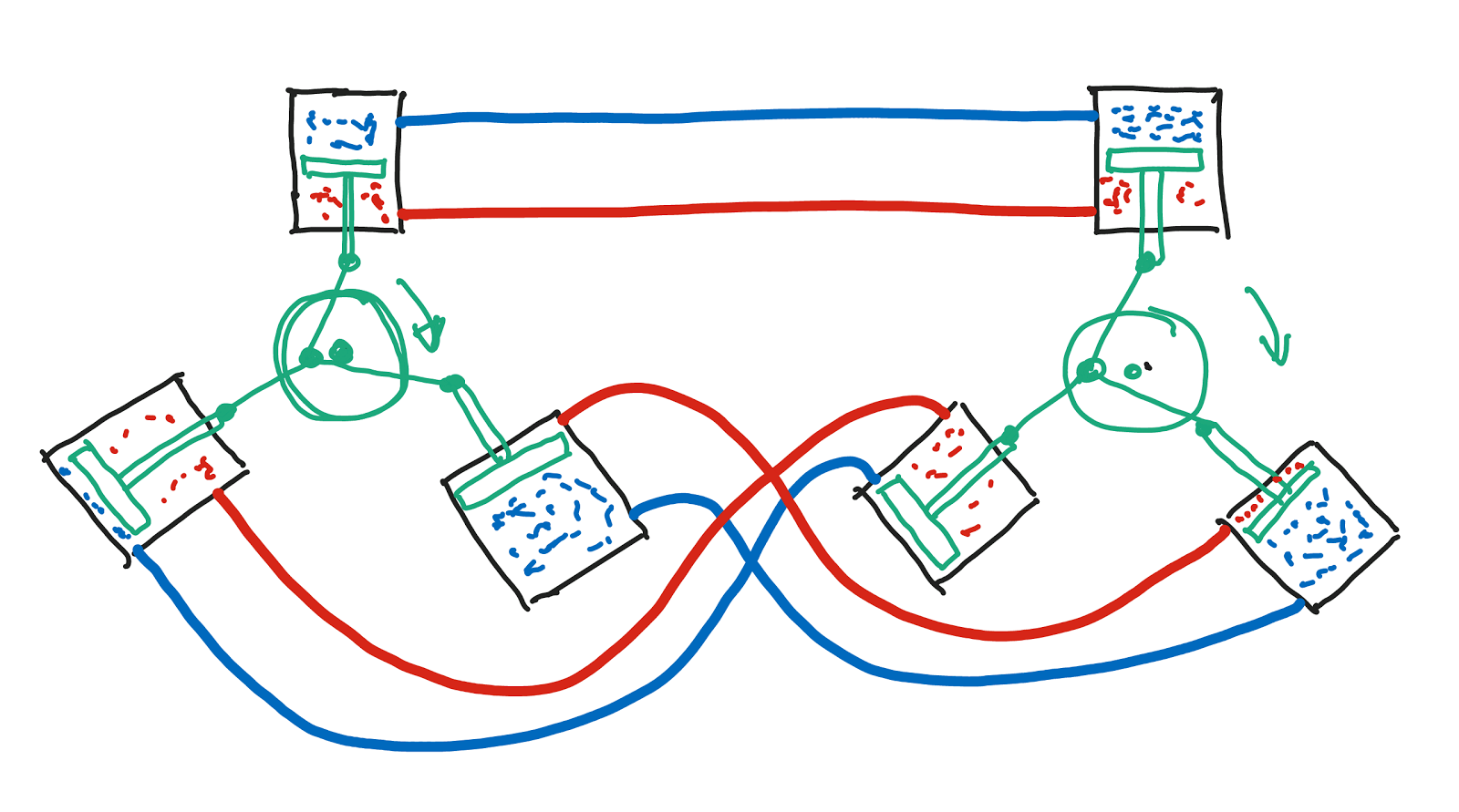You could run the three pairs in sync (water flowing at same rate and direction at any given time in all A/B/C pipes and all N1/N2/N3 pipes) or you could run them out of sync (e.g. A flowing full speed in one direction, B about to reverse and C flowing full speed in reverse). Notice that if all systems have similar flows (except at different times), when N1 flows in one direction, N2 and N3 flow in the opposite direction. Moreover, if you shift them out of sync by exactly ⅓ cycle each, the flow in the N-pipes will effectively cancel out and you don’t really need the N pipes at all (or maybe you use just one common N-pipe  instead of three to take care of any imbalances in the flow through the A-pipes that do not perfectly cancel out).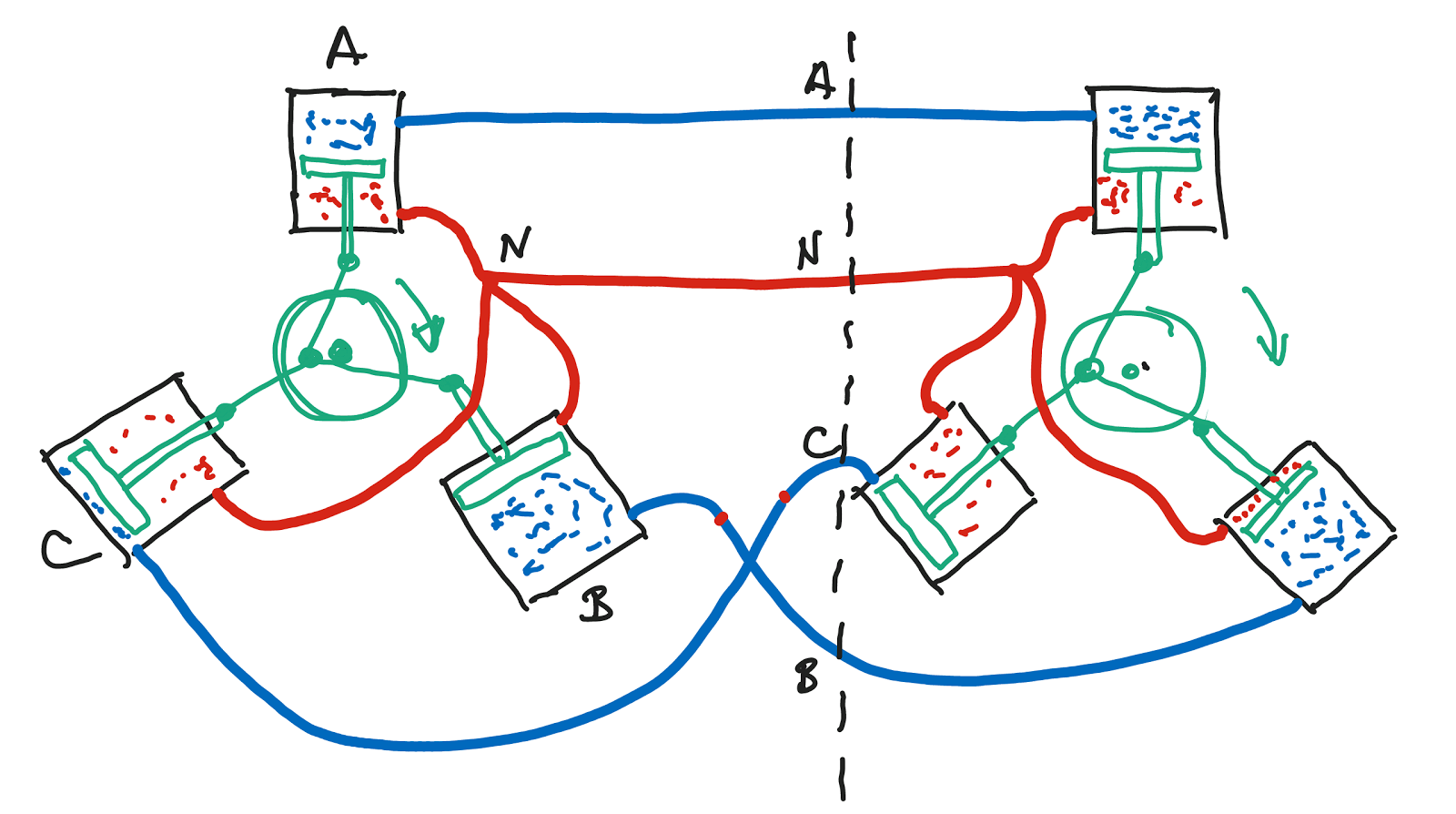Single "N" pipe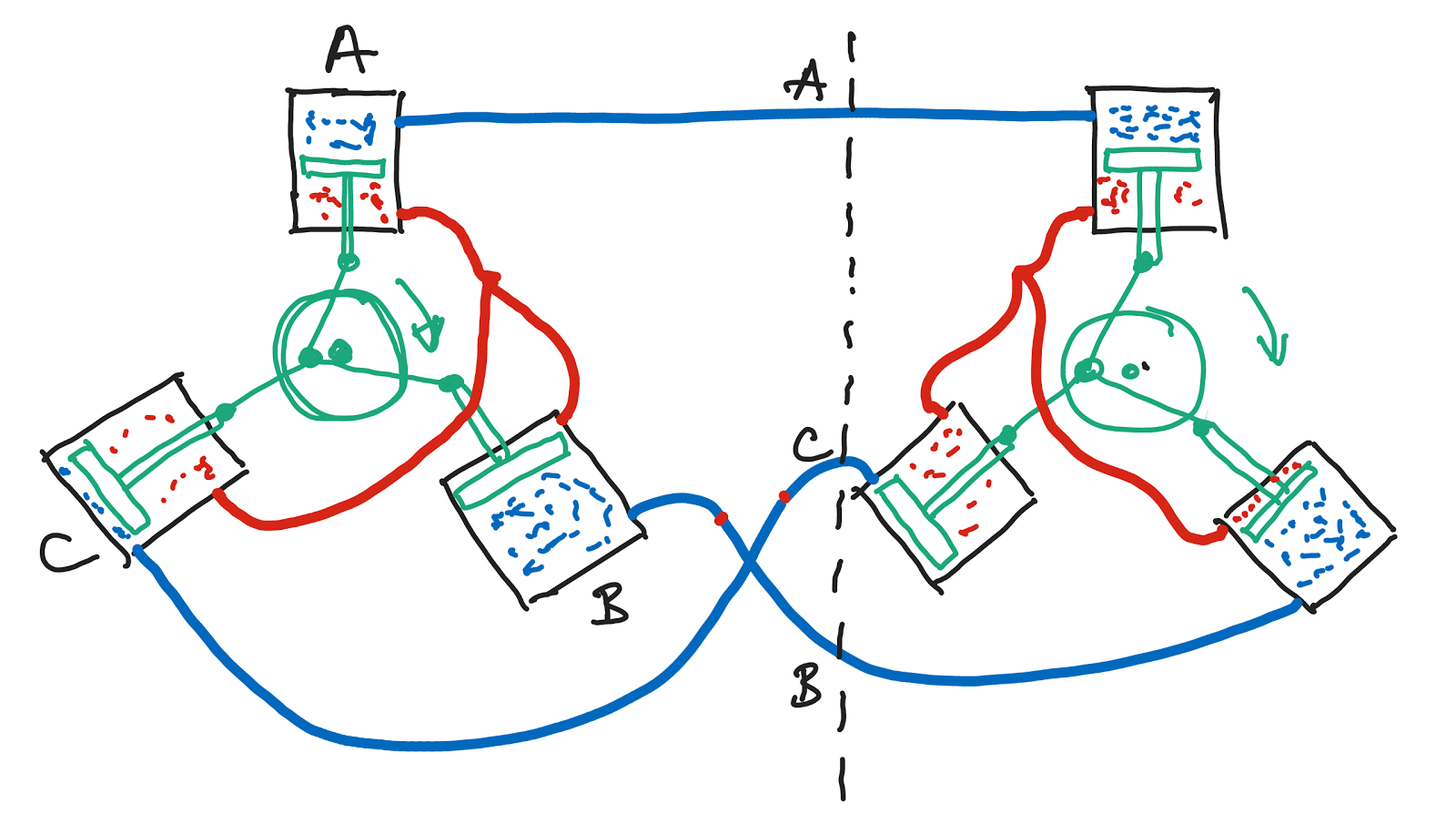No "N" pipe at all

The same idea works for three electrical circuits. That’s why 3-phase power is so popular. It lets you transfer the same amount of power with less wiring, in some cases 50% less (using 3 wires instead of 6). In order for it to work you need three synchronized power sources (the three “phases”, usually called X, Y and Z) shifted by ⅓ cycle. The common "B" pipe in this arrangement is the "neutral".

If you use only the “A pipes” it is called a “Delta” (triangle) connection.  In this configuration you are completely skipping the “B pipe” - the "neutral" magically disappears!  In a delta 3-phase connection you use 3 power-carrying conductors (generally labeled X, Y, and Z). You may also have a 4th ground wire for safety. This is what electricians call a 3-pole 3-wire connection (3P3W, without ground) or 3-pole 4-wire connection (3P4W, with ground).

If you use the three "A pipes" and a common "B pipe" it is called a Y (“Wye”) connection (three legs plus a center). In a Y connection you use 4 power carrying conductors (labeled X, Y, Z and N) and an optional 5th ground wire for safety. This is what electricians call a 4-pole 4-wire connection (4P4W, without ground) or 4-pole 5-wire connection (4P5W, with ground).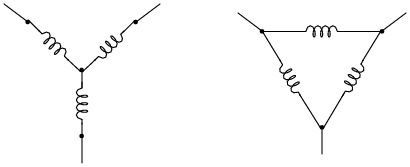3-phase power systems:  Y (Wye) and Delta

With a 3-phase supply you have two ways of connecting a traditional 2-wire load, such as a light bulb or a server. In a Y system you can connect it between any phase (X, Y or Z) and neutral (N). In both Y and Delta systems you can also connect it between any two phases (X-Y, Y-Z or Z-X).

In a 3-phase system the voltage between any two phases is 3 times higher than the voltage of an individual phase by a factor of 1.73 (square root of 3 to be exact). If your X-N (and Y-N and Z-N) voltage is 120V (common in the US), the X-Y (and Y-Z and Z-X) voltages (a.k.a. “cross-phase” voltages) will be 120V * 1.73 = 208V. The 208V (sometimes confused with the European 220V) comes from cross-phase connections to a 120V three-phase system.  A 220V system with three 220V phases has a 220 * 1.73 = 380V cross-phase voltage.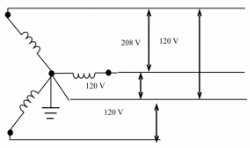Packet Power energy monitoring systems support 3-phase power in both Wye and Delta configurations and measure all key parameters of each individual phase within the circuit as well as total power and energy consumption. Send an email to if you'd like more information.

If you found this information helpful, you may also enjoy several recent blog posts.

Volts, Amps, Watts, Watt-hours and Cost

Power Factor:  The difference between promise and reality

DC in the DC